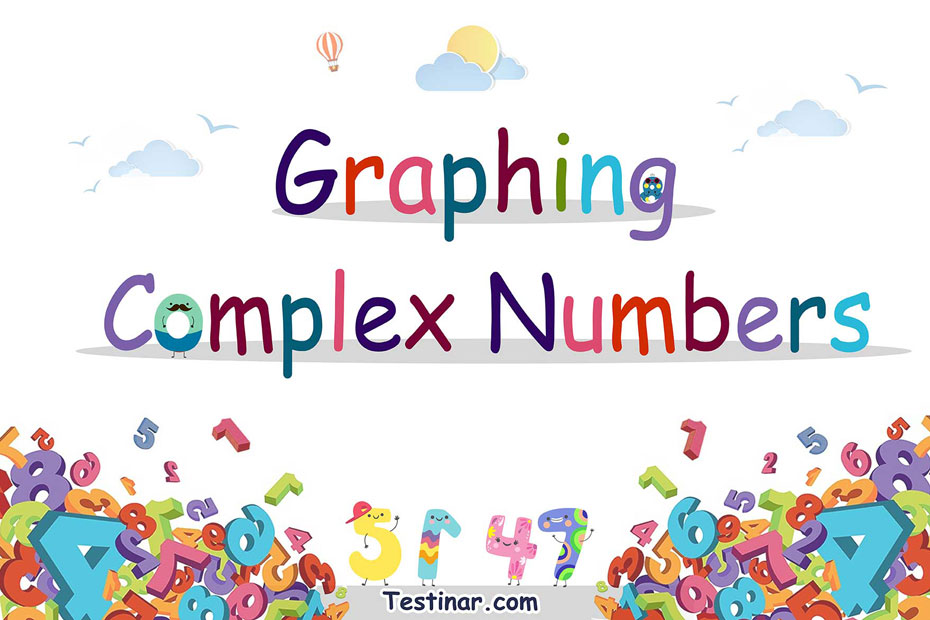## How to Graph Complex Numbers

### Graphing Complex Numbers

To plot complex numbers on the complex plane, follow the steps below.

• Find out what part of the given complex number is real and what part is imaginary. For example, the real part of $$z \ = \ a \ + \ ib$$ is $$a$$, and the imaginary part is $$b$$.
• Make a pair where the first part is real and the second part is imaginary.
For example, the ordered pair for $$z \ = \ a \ + \ ib$$ is $$(a \ , \ b)$$
• Mark on the plane the point $$(a \ , \ b)$$.

### Example

Plot $$z \ = \ 2 \ + \ 3i$$

Solution:

The real number is $$2$$, and the imaginary number is $$3$$. So, the ordered pair is $$(2 \ , \ 3)$$.

The graph below shows the complex number $$z \ = \ 2 \ + \ 3i$$: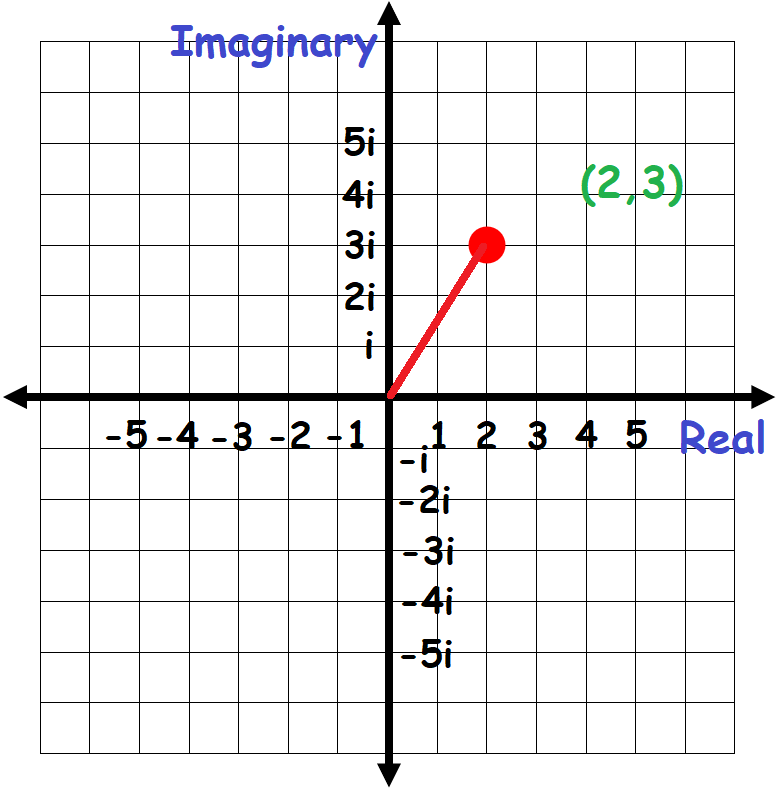### Exercises for Graphing Complex Numbers

1) Graph number in the complex plane: $$-3 \ - \ 2i$$

2) Graph number in the complex plane: $$5 \ - \ i$$

3) Graph number in the complex plane: $$5 \ + \ 3i$$

4) Graph number in the complex plane: $$-2 \ + \ 2i$$

5) Graph number in the complex plane: $$2 \ - \ 2i$$

6) Graph number in the complex plane: $$4 \ - \ i$$

7) Graph number in the complex plane: $$-3 \ - \ 5i$$

8) Graph number in the complex plane: $$-4 \ + \ 4i$$

9) Graph number in the complex plane: $$2 \ - \ 5i$$

10) Graph number in the complex plane: $$-4 \ - \ i$$

1) Graph number in the complex plane: $$-3 \ - \ 2i$$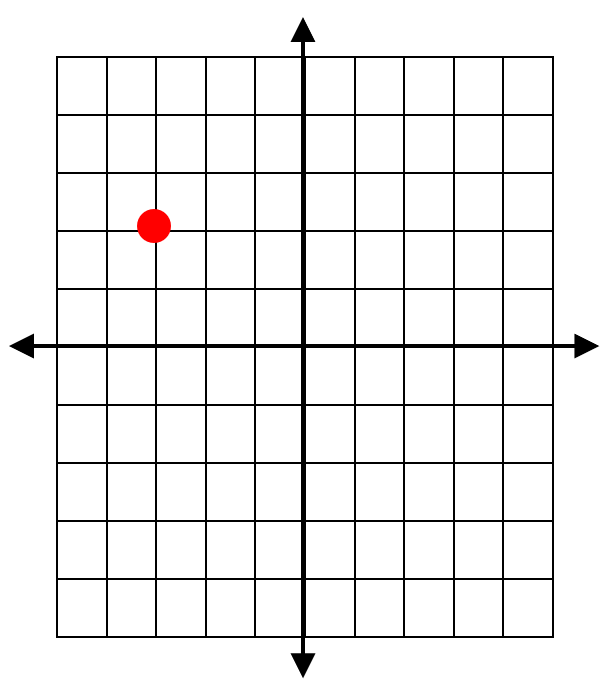2) Graph number in the complex plane: $$5 \ - \ i$$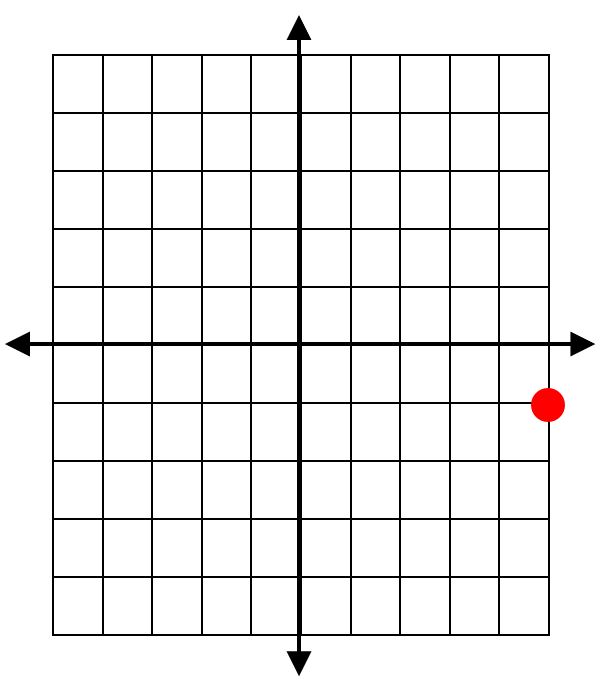3) Graph number in the complex plane: $$5 \ + \ 3i$$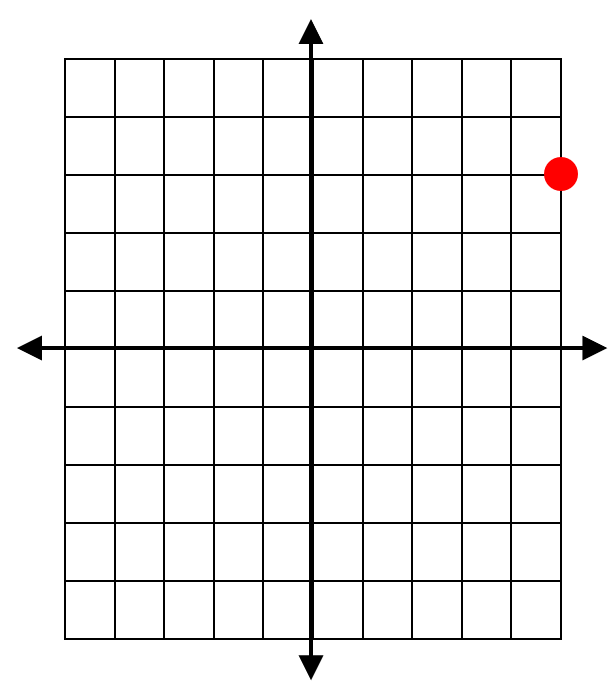4) Graph number in the complex plane: $$-2 \ + \ 2i$$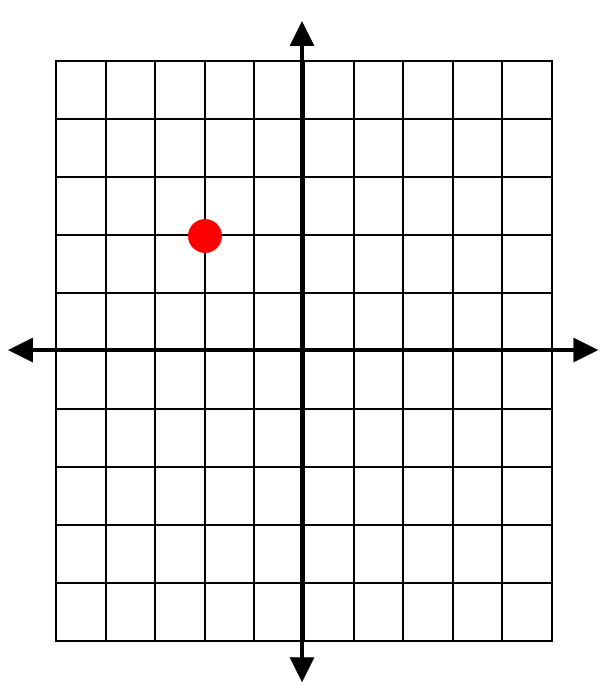5) Graph number in the complex plane: $$2 \ - \ 2i$$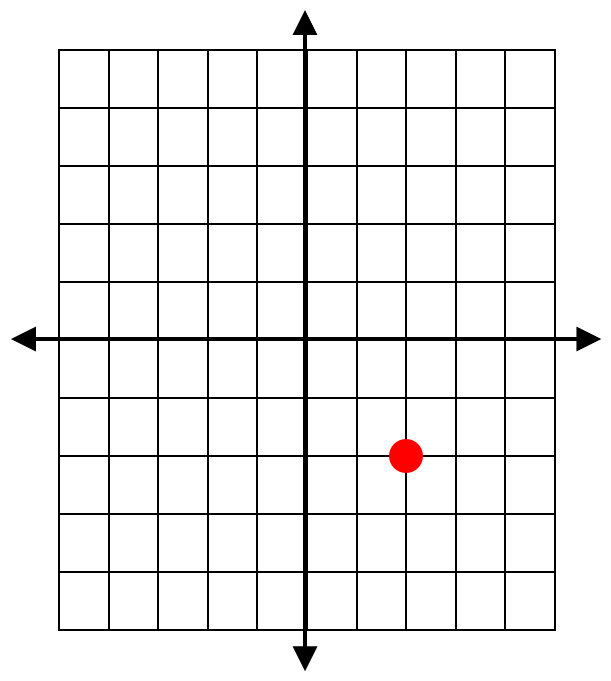6) Graph number in the complex plane: $$4 \ - \ i$$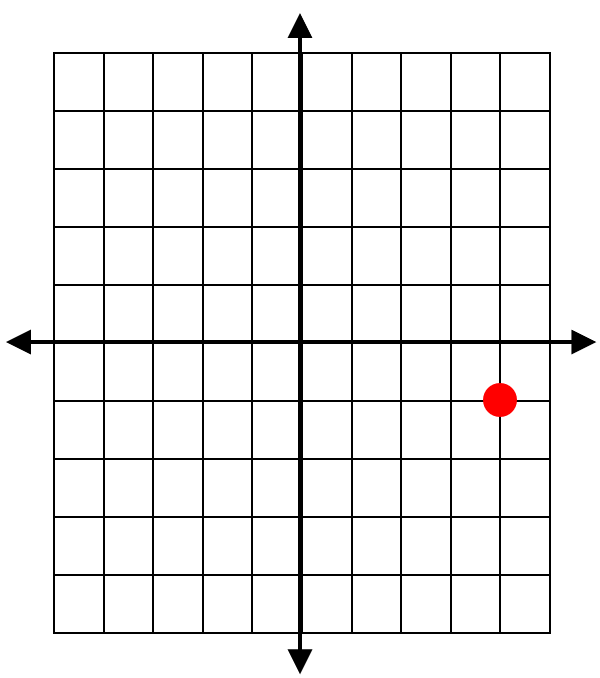7) Graph number in the complex plane: $$-3 \ - \ 5i$$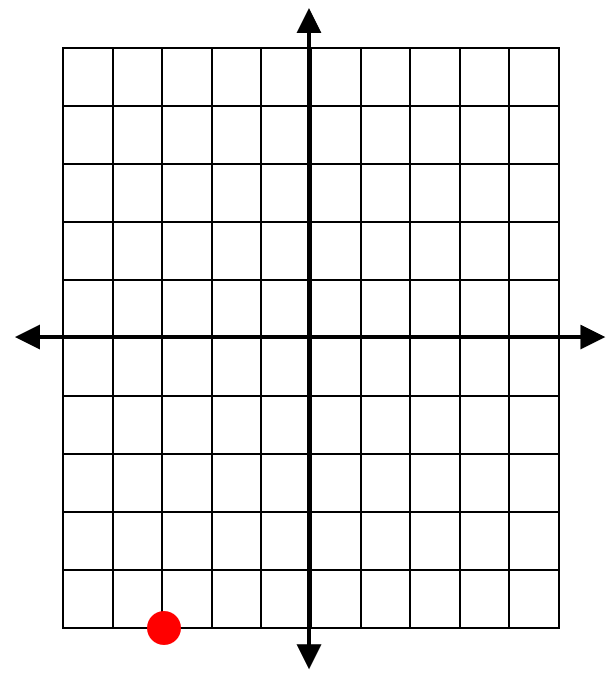8) Graph number in the complex plane: $$-4 \ + \ 4i$$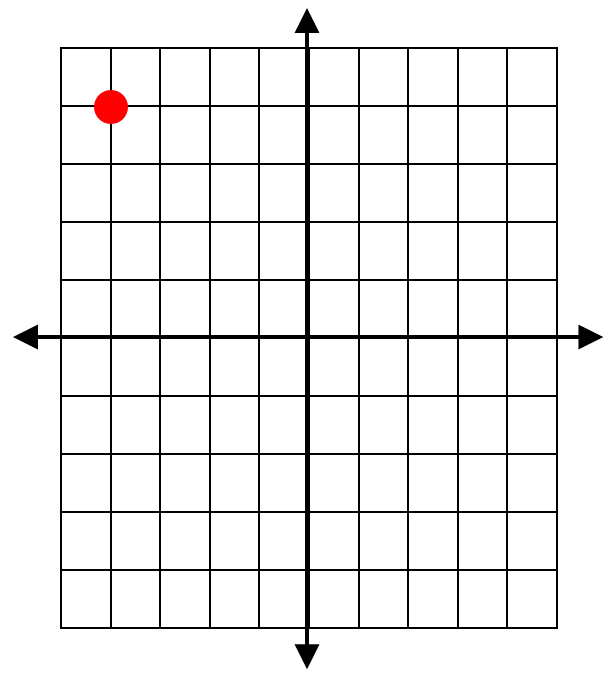9) Graph number in the complex plane: $$2 \ - \ 5i$$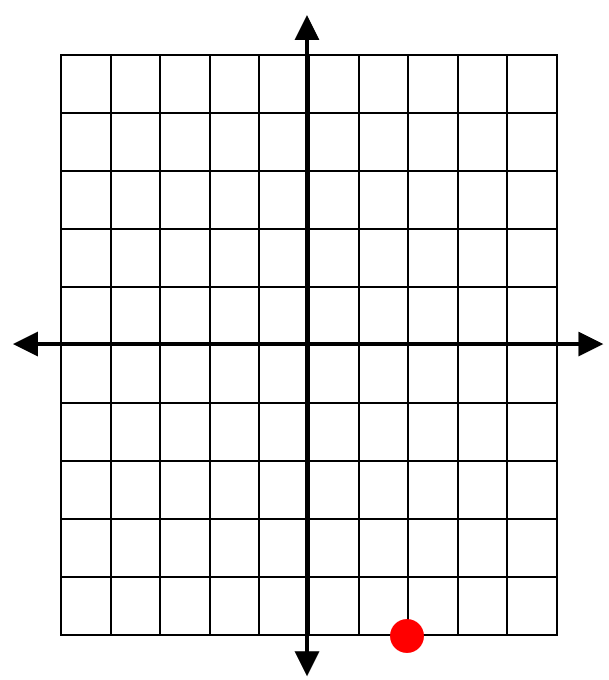10) Graph number in the complex plane: $$-4 \ - \ i$$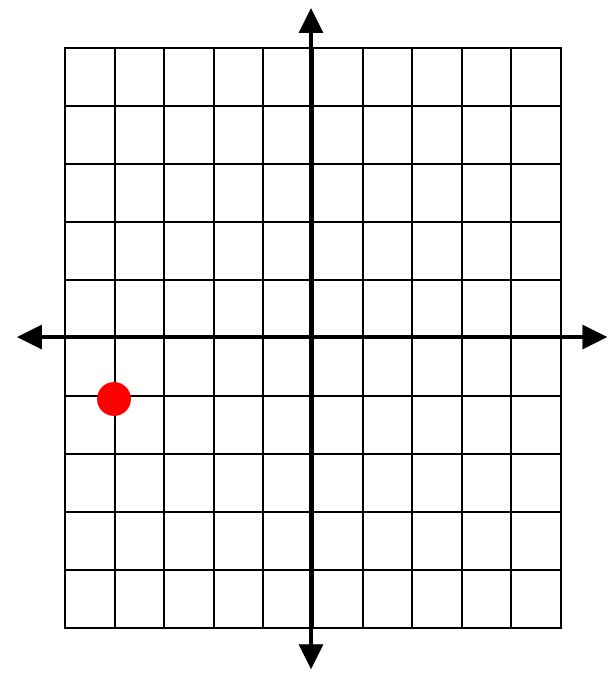## Graphing Complex Numbers Practice Quiz

### Prepare for the PSAT / NMSQT Math Test in 7 Days

$17.99$12.99

### AFOQT Math for Beginners

$24.99$14.99

### CLEP College Algebra Exercise Book

$18.99$14.99

### The Most Comprehensive ALEKS Math Preparation Bundle

$76.99$34.99